## Friday, December 10, 2010

### 22.7: Crystal Field Theory

Crystal Field Theory:
Remember what the 3d orbitals look like:Simplify the ligands into negatively charged or slightly negatively charged point particles. A complex ion with six ligands will have them arranged octahedrally around the metal atom (because they will repel each other). The arrangement of these negative charges will lie on the x, y, and z axes, as represented by the red spheres below: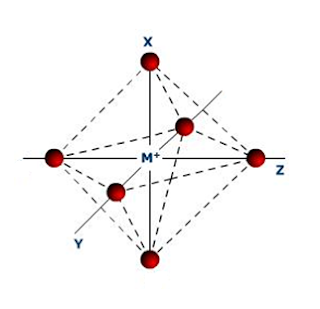Notice that the arms of the dz2 and dx2 – y2 orbitals also lie along the axes where the octahedrally arranged ligands (negative point particles) lie (the other orbitals lie between the axes). Those electrons will have a stronger repulsive effect on the ligands, creating a situation where the five 3d orbitals are no longer of the same energy.

These two orbitals that lie on the axes will have higher energy, and the other three will have lower energy.Where Δ = crystal field splitting = the difference in energy between the two sets of d orbitals on a central metal ion that arises from the interaction of the orbitals with the electric field of the ligands.

High and Low Spin Complexes:

Pairing Energy: the energy required to put two electrons into the same orbital, denoted P. When an orbital already has an electron in it, there needs to be an input of energy to overcome the repulsion these two electrons will have for each other, this is the pairing energy.

Imagine the electron configuration of a metal ion in a complex, specifically the 3d orbitals. There are five to fill. Three have lower energy than the other two, which have higher energy. So the three low energy orbitals get filled first. But say there are four electrons in the configuration, not three, how do we know whether to start pairing the electrons in the low energy orbitals or to go ahead and start filling the empty, higher energy orbitals?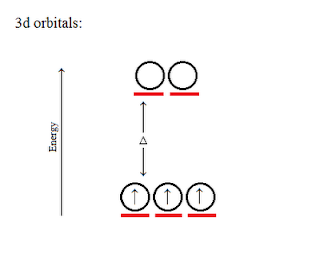It depends on P and Δ. If P is greater than Δ, this means it requires more energy to pair an unpaired electron in a lower energy orbital than it requires to boost that electron up to the higher energy orbital. So, if P is greater than Δ, that fourth electron goes in the first empty high energy orbital, and it is called a high spin complex: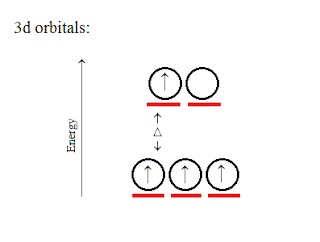If Δ is greater than P, this means it requires more energy to boost that electron up to the higher energy orbital than it does to pair an unpaired electron in a lower energy orbital. So, if Δ is greater than P, that fourth electron pairs with the first unpaired electron in the low energy orbital, and it is called a low spin complex: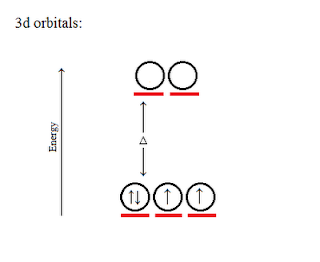Here is a complete filling of the 3d orbitals, comparing high spin and low spin:Paramagnetic substances have at least one unpaired electron in their orbitals. Most of the configurations in the diagram above show paramagnetism. Diamagnetic substances have no unpaired electrons in their orbitals. Diamagnetism is shown in the diagram above at six electrons at low spin, and at ten electrons at both high and low spin.

Spectrochemical Series: an arrangement of ligands according to the relative magnitudes of crystal field splittings they induce in the d orbitals of a metal ion.

As Δ increases, ligands bond more strongly (found on page 957).

Example:
Which one is high spin, [CrBr6]3– or [Cr(CN)6]3–?

From the spectrochemical series, we see that Br has a relatively small Δ, so it is high spin.
CN has a relatively large Δ, so it is low spin.

Visible Spectra of Transition-Metal Complexes:

Many transition-metal substances are colored, and the color is related to Δ through the wavelength, λ, of light absorbed:

Δ = hυ = hc / λ
or
λ = hc / Δ

where:
Δ = crystal field splitting
λ = wavelength
υ = frequency
h = Planck's Constant = 6.626068 x 10-34 J · s
c = the speed of light = 3.00 x 108 m / s
Note: With these problems, wavelength might be given in nanometers, but the rest of the units are in meters. To convert: 1 nm = 1 x 10–9 m

Example:
For a certain substance, if λ = 555 nm, what is Δ?

Convert from nanometers to meters: 555 nm = 555 x 10–9 m = λ
h = 6.626068 x 10-34 J · s
c = 3.00 x 108 m / s

Δ = hc / λ
Δ = [(6.626068 x 10-34 J · s)(3.00 x 108 m / s)] / (555 x 10–9 m)
Δ = 3.58 x 10-19J

Example:
For a certain substance, if Δ = 2.00 x 10–19, what is λ?

λ = hc / Δ
λ = [(6.626068 x 10-34 J · s)(3.00 x 108 m / s)] / (2.00 x 10–19 J)
λ = 9.95 x 10–7 m = 995 nm

1.hai sir. let me introduce myself. i'm fatim neni. i juz want know how's bout energy diagram for 3d orbitals for tetrahedral. could you post it coz i will sitting for my final examination next week. thank you.

2.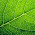I recently sold the book I used for this class, so I'm not sure that I am able to answer your question, but I did find this: "In a tetrahedral crystal field splitting the d-orbitals again split into two groups, with an energy difference of Δtet where the lower energy orbitals will be dz2 and dx2-y2, and the higher energy orbitals will be dxy, dxz and dyz - opposite to the octahedral case. Furthermore, since the ligand electrons in tetrahedral symmetry are not oriented directly towards the d-orbitals, the energy splitting will be lower than in the octahedral case." From here. I hope this is helpful.

(Also, I'm a woman, not a sir. Not a big deal.)

3.